GeeksforGeeks App
Open AppBrowser
Continue

# Introduction of Algorithmic State Machines (ASMs)

Introduction :

• In digital systems, the binary information is divided into data and control information.
• Data information is manipulated by various operations like arithmetic, shift, and logic. These operations are implemented by multiplexers, decoders, counters, and shift registers.
• Control information gives various command signals that help to perform various data operations.
• Therefore, the logic design of digital systems can be divided into two parts in which one part is responsible for design of the circuit that performs data processing operations and the other part is responsible for design of the control circuit that takes care of all the operations and their sequence.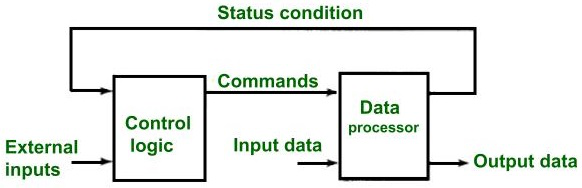Relationship between control logic and data processor

• The control sequence and data processing of the digital system can be determined by a hardware algorithm. So our motive is to formulate the hardware algorithm that helps to achieve our objective.
• A flow chart is a traditional method for determining the sequence of procedural steps and decision paths for a hardware algorithm.
• A special type of flow chart is developed to specify the design of a digital circuit also known as an Algorithmic state machine(ASM) chart.

ASM Chart :
It is a special type of flow chart that is used to describe the sequential operations of a digital circuit. The ASM chart determines the sequence of events, timing relationship between the states of sequential controller ,and the events that happen while going from one state to another.

The ASM chart is composed of three basic elements, which are

• State box
• Decision box
• Conditional box

State box :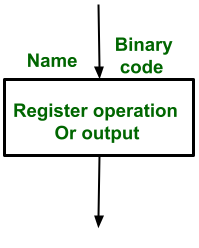State box

• A state in the control sequence is described by State box.
• The shape of the state box is rectangular in which register operations or output signal names can be specified.
• The state name is given a symbolic name which is written in the upper left corner of the box.
• After the state assignment, the binary code is placed at upper right corner of the box.

Decision box :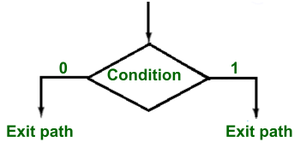Decision box

• It describes the effect of the input on the control subsystem.
• It is a diamond-shaped box with two or more exit paths. The input condition which needs to be checked is written inside the box.
• One exit path is taken when the condition is true, otherwise other is taken when the condition is false.
• When the input condition is assigned to a binary value, then the two paths are indicated by 1 and 0

Condition box :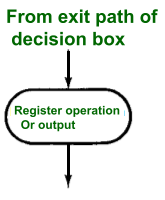Condition box

• It has an oval shape. The input path of the conditional box must come from the exit path of the decision box.
• The register operations and output lists are written inside the conditional box which is generated in a particular state but the input condition must be true.

Example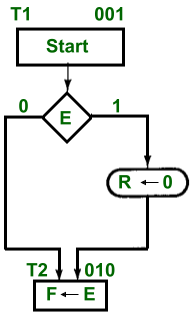• The control(i.e. control unit) generates Start output signal when T1 state arises.
• In T1, control checks the status of input E.
• If E= 1, then R is assigned to 0, otherwise R remains unchanged. In any value of E the next state will be T2.

ASM block :

• An ASM block contains one state box and all the decision boxes and conditional boxes connected to its exit path.
• It contains one entrance path and one or more exit paths.

Example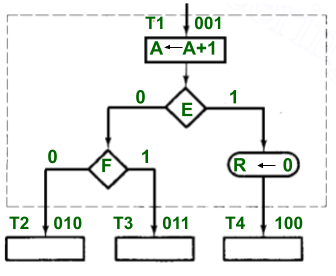ASM block

• The block(dashed lines) is associated with T1 state and has two decisions and one conditional box. Each block in the ASM determines the state of the system during one clock pulse. The operations within the state and conditional boxes are executed in the common clock pulse( i.e. in T1 state).This clock pulse also changes the state of the system (i.e. The other state could be T2 or T3 or T4), which can be known by the binary values of the decision boxes E and F.
• In state T1, a register value is incremented by 1.
• If E= 1, then the register R is reset.
• Here, each state box is equivalent to the state in a sequential circuit.
My Personal Notes arrow_drop_up